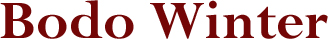Here, you can find tutorials on various aspects of statistics and R. The tutorials are meant to be super-short and accessible. If you like them, consider my two- or three-day workshop on mixed models that goes into much more depth.

If you're interested in getting automatic updates on tutorials, enter your email into this form:

# Statistics Tutorials

Linear Models and Mixed Models with R

This is a decidedly conceptual introduction to the linear model framework and linear mixed effects models in R. It is intended to be very basic.

tutorial 1 pdf
tutorial 2 pdf        dataset for tutorial 2

Winter, B. (2013). Linear models and linear mixed effects models in R with linguistic applications. arXiv:1308.5499. [http://arxiv.org/pdf/1308.5499.pdf]

Understanding the F distribution and the principle behind ANOVAs

F-values, the F-distribution, sum of squares ... these are concept you struggle with? Maybe this tutorial helps!